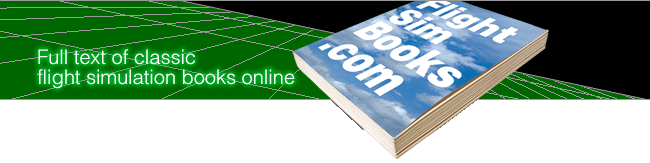Airienteering with 13MIKE

by Fred J. Calfior and Douglas W. Miller

AirLeg 2

1) Intercept and fly counterclockwise on the SFO VOR “20.0” DME arc

2) When overhead “COLLI” intersection, press “P” to pause the simulation

Record your:

Altitude_________________________________(45 pts)

DME from OAK VOR_______________________(35 pts)

VSI ____________________________________(20 pts)

3) Press “P” to continue the simulation

4) How many VFR Sectional charts are used to cover the continental United States?

Go to AirLeg 15 if the right answer is:

The number obtained by taking 15, subtract the number of years in a decade, and square the result!

Go to AirLeg 48 if the right answer is:

The number obtained by taking 48, reverse the numbers, divide by 7, multiply it by the sum of its digits, then add 1!

Go to AirLeg 43 if the right answer is:

The number obtained by taking 70, divide it by 8, take the remainder and multiply it by 10% of the original number!